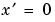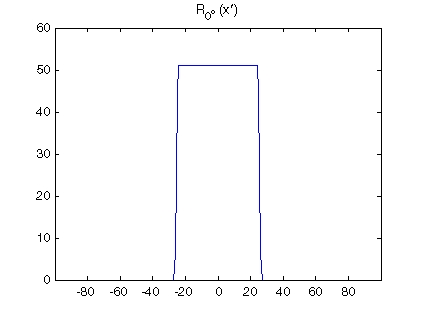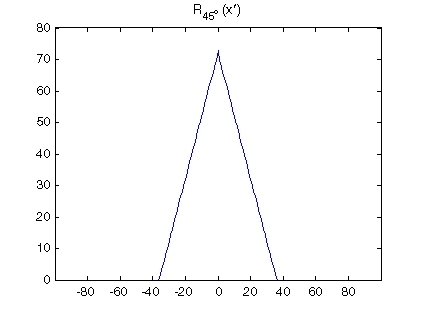Image Processing Toolbox User's GuideYou can compute the Radon transform of an image I for the angles specified in the vector theta using the radon function with this syntax.

• [R,xp] = radon(I,theta);


The columns of R contain the Radon transform for each angle in theta. The vector xp contains the corresponding coordinates along the x´-axis. The center pixel of I is defined to be floor((size(I)+1)/2); this is the pixel on the x´-axis corresponding to.

The commands below compute and plot the Radon transform at 0° and 45° of an image containing a single square object. xp is the same for all projection angles.

• I = zeros(100,100);
I(25:75, 25:75) = 1;
imshow(I)figure; plot(xp,R(:,1)); title('R_{0^o} (x\prime)')Radon Transform of a Square Function at 0 Degrees

• figure; plot(xp,R(:,2)); title('R_{45^o} (x\prime)')Radon Transform of a Square Function at 45 DegreesRadon Transform Viewing the Radon Transform as an Image